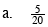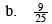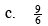### Home > MC2 > Chapter 1 > Lesson 1.2.3 > Problem1-78

1-78.Can you reduce this fraction?

What is a common factor of both 5 and 20?

A percent is a portion out of 100. Set the fraction as an equivalent fraction out of 100.Refer to part (a).

$\frac{18}{50}\text{ or } 36\%$Reduce this fraction to find an equivalent fraction. Then find an equivalent fraction out of 100 to get the percent.